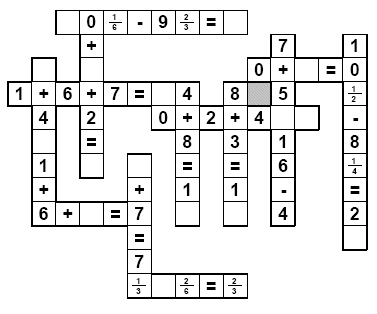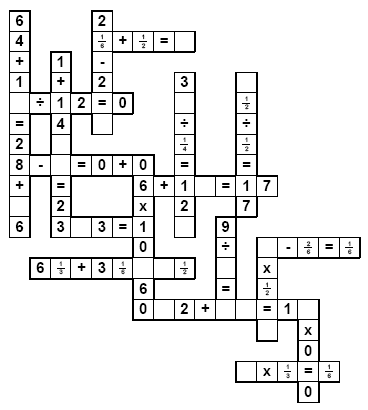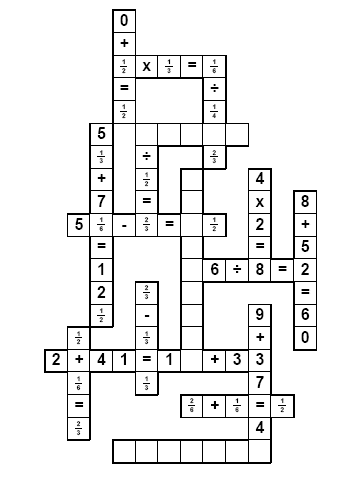Worksheets and No Prep Teaching Resources Runaway Math UnitRunaway Mathwith Fractions

Runaway Math Puzzles
Fifth and sixth grade runaway math puzzles

Runaway Math Puzzles: Third Grade - With FractionsAddition - Missing All the Numbers in Equations
Fractions: One equation missing (only numbers)
Fractions: Two equations missing (only numbers)
Fractions: Three equations missing (only numbers)
Fractions: One equation missing
Fractions: Two equations missing
Fractions: Three equations missing
Addition - Missing Numbers (smaller puzzle)
Fractions: Three numbers missing
Fractions: Five numbers missing
Fractions: Seven numbers missing
Fractions: Three numbers missing and all of the operations missing
Addition - Missing Numbers (larger puzzle)
Fractions: Five numbers missing
Fractions: Seven numbers missing
Fractions: Nine numbers missing
Addition - Missing Pieces (may be a number or a symbol)
Fractions: Three pieces missing
Fractions: Five pieces missing
Fractions: Seven pieces missing
Addition - Missing Pieces (may be a number or a symbol; larger puzzle)
Fractions: Five pieces missing
Fractions: Seven pieces missing
Fractions: Nine pieces missing
Fractions: 20 % of puzzle missing (smaller puzzle)
Fractions: 40 % of puzzle missing (smaller puzzle)
Fractions: 20 % of puzzle missing
Fractions: 40 % of puzzle missing

Subtraction - Missing All the Numbers in Equations
Fractions: One equation missing (only numbers)
Fractions: Two equations missing (only numbers)
Fractions: Three equations missing (only numbers)
Subtraction - Missing Entire Equations
Fractions: One equation missing
Fractions: Two equations missing
Fractions: Three equations missing
Subtraction - Missing Numbers (smaller puzzle)
Fractions: Three numbers missing
Fractions: Five numbers missing
Fractions: Seven numbers missing
Fractions: Three numbers missing and all of the operations missing
Subtraction - Missing Numbers (larger puzzle)
Fractions: Five numbers missing
Fractions: Seven numbers missing
Fractions: Nine numbers missing
Subtraction - Missing Pieces (may be a number or a symbol)
Fractions: Three pieces missing
Fractions: Five pieces missing
Fractions: Seven pieces missing
Subtraction - Missing Pieces (may be a number or a symbol; larger puzzle)
Fractions: Five pieces missing
Fractions: Seven pieces missing
Fractions: Nine pieces missing
Subtraction - Missing Pieces
Fractions: 20 % of puzzle missing (smaller puzzle)
Fractions: 40 % of puzzle missing (smaller puzzle)
Fractions: 20 % of puzzle missing
Fractions: 40 % of puzzle missing

Addition and Subtraction - Missing All the Numbers in Equations
Fractions: One equation missing (only numbers)
Fractions: Two equations missing (only numbers)
Fractions: Three equations missing (only numbers)
Addition and Subtraction - Missing Entire Equations
Fractions: One equation missing
Fractions: Two equations missing
Fractions: Three equations missing
Addition and Subtraction - Missing Numbers (smaller puzzle)
Fractions: Three numbers missing
Fractions: Five numbers missing
Fractions: Seven numbers missing
Fractions: Three numbers missing and all of the operations missing
Addition and Subtraction - Missing Numbers (larger puzzle)
Fractions: Five numbers missing
Fractions: Seven numbers missing
Fractions: Nine numbers missing
Addition and Subtraction - Missing Pieces (may be a number or a symbol)
Fractions: Three pieces missing
Fractions: Five pieces missing
Fractions: Seven pieces missing
Addition and Subtraction - Missing Pieces (may be a number or a symbol; larger puzzle)
Fractions: Five pieces missing
Fractions: Seven pieces missing
Fractions: Nine pieces missing
Addition and Subtraction - Missing Pieces
Fractions: 20 % of puzzle missing (smaller puzzle)
Fractions: 40 % of puzzle missing (smaller puzzle)
Fractions: 20 % of puzzle missing
Fractions: 40 % of puzzle missing

Runaway Math Puzzles: Fourth Grade - With FractionsFractions: Five numbers missing
Fractions: Seven numbers missing
Fractions: Nine numbers missing
Fractions: Two equations missing
Fractions: Three equations missing
Fractions: Seven numbers or operators missing
Fractions: Nine numbers or operators missing
Fractions: 20 % of puzzle missing
Fractions: 20 % of puzzle missing and all operators
Fractions: 40 % of puzzle missing
Fractions: Everything missing except for operators (very challenging)
Fractions: Seven numbers missing
Fractions: Nine numbers missing
Fractions: Eleven numbers missing
Fractions: Two equations missing
Fractions: Three equations missing
Fractions: Seven numbers or operators missing
Fractions: Nine numbers or operators missing
Fractions: Twelve numbers or operators missing
Fractions: 20 % of puzzle missing
Fractions: 20 % of puzzle missing and all operators
Fractions: 40 % of puzzle missing

Easy Multiplication and Division Puzzles
Runaway math for third grade has easier puzzles for multiplication and division
Multiplication and Division
Fractions: Five numbers missing
Fractions: Seven numbers missing
Fractions: Nine numbers missing
Fractions: Two equations missing
Fractions: Three equations missing
Fractions: Seven numbers or operators missing
Fractions: Nine numbers or operators missing
Fractions: 20 % of puzzle missing
Fractions: 20 % of puzzle missing and all operators
Fractions: 40 % of puzzle missing
Fractions: Everything missing except for operators (very challenging)
Multiplication and Division (more challenging)
Fractions: Seven numbers missing
Fractions: Nine numbers missing
Fractions: Eleven numbers missing
Fractions: Two equations missing
Fractions: Three equations missing
Fractions: Seven numbers or operators missing
Fractions: Nine numbers or operators missing
Fractions: Twelve numbers or operators missing
Fractions: 20 % of puzzle missing
Fractions: 20 % of puzzle missing and all operators
Fractions: 40 % of puzzle missing

Fractions: Five numbers missing
Fractions: Seven numbers missing
Fractions: Nine numbers missing
Fractions: Two equations missing
Fractions: Three equations missing
Fractions: Seven numbers or operators missing
Fractions: Nine numbers or operators missing
Fractions: 20 % of puzzle missing
Fractions: 20 % of puzzle missing and all operators
Fractions: 40 % of puzzle missing
Fractions: Everything missing except for operators (very challenging)
Addition, Subtraction, Multiplication, and Division (more challenging)
Fractions: Seven numbers missing
Fractions: Nine numbers missing
Fractions: Eleven numbers missing
Fractions: Two equations missing
Fractions: Three equations missing
Fractions: Seven numbers or operators missing
Fractions: Nine numbers or operators missing
Fractions: Twelve numbers or operators missing
Fractions: 20 % of puzzle missing
Fractions: 20 % of puzzle missing and all operators
Fractions: 40 % of puzzle missing

Removing Two Numbers from Equations - With FractionsFractions: All of the equations are each missing at least one number (easy; most are automatic)
Fractions: Large puzzle with three missing equations
Fractions: Ten missing pieces
Fractions: Fifteen missing pieces
Fractions: Three of the equations are each missing at least two numbers
Fractions: All of the equations are each missing at least two numbers (difficult)
Fractions: All of the equations are each missing at least two numbers (larger puzzle; even more difficult!)
Fractions: Everything missing except for operators (very challenging; as some may say "good luck!")

Multiplication and Division Puzzles
Fractions: All of the equations are each missing at least one number (easy; most are automatic)
Fractions: Large puzzle with three missing equations
Fractions: Ten missing pieces
Fractions: Fifteen missing pieces
Fractions: Three of the equations are each missing at least two numbers
Fractions: All of the equations are each missing at least two numbers (difficult)
Fractions: All of the equations are each missing at least two numbers (larger puzzle; even more difficult!)
Fractions: Everything missing except for operators (very challenging; as some may say "good luck!")

Addition, Subtraction, Multiplication, and Division Puzzles
Fractions: All of the equations are each missing at least one number (easy; most are automatic)
Fractions: Large puzzle with three missing equations
Fractions: Ten missing pieces
Fractions: Fifteen missing pieces
Fractions: Three of the equations are each missing at least two numbers
Fractions: All of the equations are each missing at least two numbers (difficult)
Fractions: All of the equations are each missing at least two numbers (larger puzzle; even more difficult!)
Fractions: Everything missing except for operators (very challenging; as some may say "good luck!")

Runaway Math Puzzles: Extra pieces given on second page - With Fractions
(students will need to ignore some of the extra pieces)

Addition and Subtraction Puzzles (with extra pieces)
Fractions: Five numbers missing (one extra piece)
Fractions: Seven numbers missing (one extra piece)
Fractions: Nine numbers missing (two extra pieces)
Fractions: Two equations missing (one extra piece)
Fractions: Three equations missing (two extra pieces)
Fractions: Seven numbers or operators missing (one extra piece)
Fractions: Nine numbers or operators missing (one extra piece)
Fractions: 20 % of puzzle missing (one extra piece)
Fractions: 20 % of puzzle missing and all operators (one extra piece)
Fractions: 40 % of puzzle missing (two extra pieces)

Multiplication and Division Puzzles (with extra pieces)
Fractions: Five numbers missing (one extra piece)
Fractions: Seven numbers missing (one extra piece)
Fractions: Nine numbers missing (two extra pieces)
Fractions: Two equations missing (one extra piece)
Fractions: Three equations missing (two extra pieces)
Fractions: Seven numbers or operators missing (one extra piece)
Fractions: Nine numbers or operators missing (one extra piece)
Fractions: 20 % of puzzle missing (one extra piece)
Fractions: 20 % of puzzle missing and all operators (one extra piece)
Fractions: 40 % of puzzle missing (two extra pieces)

Addition, Subtraction, Multiplication, and Division Puzzles (with extra pieces)
Fractions: Five numbers missing (one extra piece)
Fractions: Seven numbers missing (one extra piece)
Fractions: Nine numbers missing (two extra pieces)
Fractions: Two equations missing (one extra piece)
Fractions: Three equations missing (two extra pieces)
Fractions: Seven numbers or operators missing (one extra piece)
Fractions: Nine numbers or operators missing (one extra piece)
Fractions: 20 % of puzzle missing (one extra piece)
Fractions: 20 % of puzzle missing and all operators (one extra piece)
Fractions: 40 % of puzzle missing (two extra pieces)

Teacher Recommendations:  Worksheet and Lesson Plan Activity Ideas

Math

Science

Word Search

Solar System

Plants

Poetry

Weather

Time

Handwriting

Money

Spelling

Graphic Organizers

Probability

More Activities, Lesson Plans, and Worksheets

Have a suggestion or would like to leave feedback?